# The Cantellated Tesseract

The cantellated tesseract is a uniform polytope bounded by 56 cells (8 rhombicuboctahedra, 32 triangular prisms, 16 octahedra), 248 polygons (128 triangles, 120 squares), 288 edges, and 96 vertices.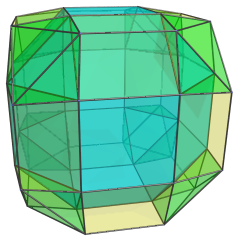## Structure

We shall explore the structure of the cantellated tesseract by means of its parallel projection into 3D, centered on a rhombicuboctahedron.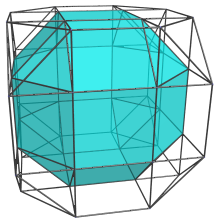The nearest cell, a rhombicuboctahedron, is shown in cyan. Twelve of its square faces are joined to triangular prisms, shown in yellow below: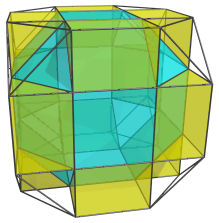Its 8 triangular faces are joined to octahedra, shown in green below: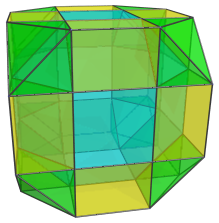These are all the cells on the “northern hemisphere” of the cantellated tesseract. The remaining 6 square faces of the nearest rhombicuboctahedron are joined to 6 other rhombicuboctahedra on the “equator”, as shown below: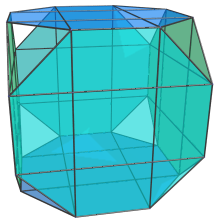For clarity, we omit the cells previously seen. These 6 rhombicuboctahedra have been foreshortened into octagons because they are lie at a 90° angle to the 4D viewpoint. They are actually perfectly uniform rhombicuboctahedra.

The triangular gaps visible here are filled in by 8 more triangular prisms, shown below in yellow: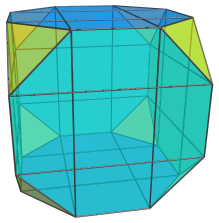As with the equatorial rhombicuboctahedra, these triangular prisms have been foreshortened into triangles because they are seen at a 90° angle. They are uniform triangular prisms in 4D.

Past this point, the cells in the “northern hemisphere” of the cantellated tesseract repeat themselves in reverse order, forming the cells of the “southern hemisphere”.

Thus, in summary, we have 1 rhombicuboctahedron from the northern hemisphere, 6 from the equator, and 1 more from the southern hemisphere, making a total of 1+6+1=8 rhombicuboctahedra. For triangular prisms, we have 12+8+12=32, and for octahedra 8+0+8=16.

## Other Projections

The following sequence of images shows the structure of the cantellated tesseract in a slightly different parallel projection: one centered on an octahedron.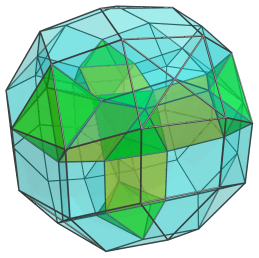For clarity, only 5 octahedra and 4 triangular prisms are shown. The octahedron in the center is the closest to the 4D viewpoint. Four of its faces are joined to the 4 triangular prisms. The other four are joined to 4 rhombicuboctahedra, as shown below: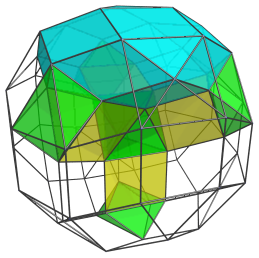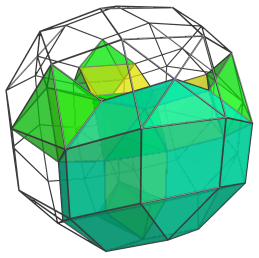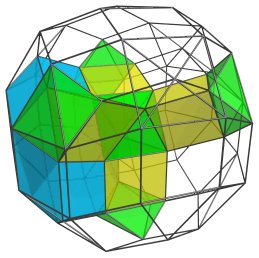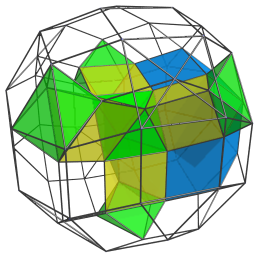Here are all 4 rhombicuboctahedra together: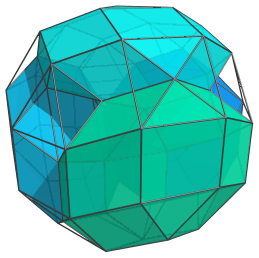And here is the network of octahedra and triangular prisms that fills in the gaps between these rhombicuboctahedra, including the 9 cells we saw at the beginning: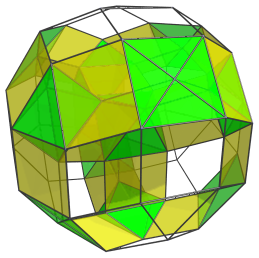Six of the octahedra lie on the “equator” in this projection, and so appear flattened into squares. They are, of course, perfectly uniform in 4D. They are shown separately below: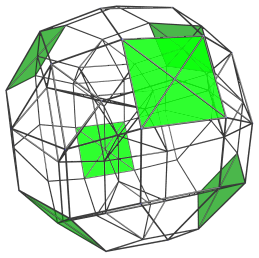## Geometric Relations

The cantellated tesseract can be constructed as an augmentation of the 8,8-duoprism by 8 square magnabicupolic rings, wedge-shaped CRF polychora whose square pyramid cells merge into the 16 regular octahedra.

These segments are attached to the 8,8-duoprism by their octagonal prism cells; they can be cut off and glued back on rotated by 45° in the plane of the octagonal faces. The result is a gyration of the cantellated tesseract. Each segment can be independently gyrated, and all gyrations of the cantellated tesseract are CRF polychora. Gyrations of interest include:

## Coordinates

The Cartesian coordinates of a cantellated tesseract with edge length 2, centered on the origin, are all permutations of coordinates and changes of sign of:

• (1, 1, (1+√2), (1+√2))

Last updated 17 Jun 2019.